# Prime Factor Calculator

10K+Everyone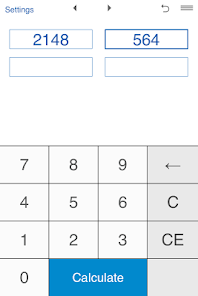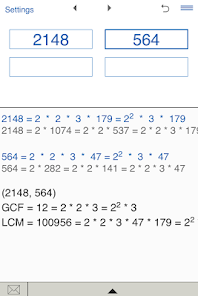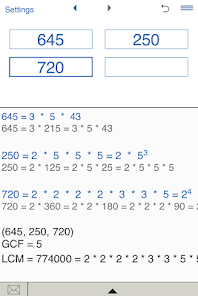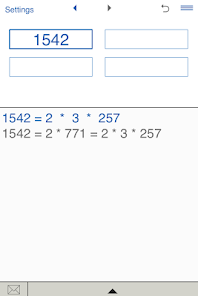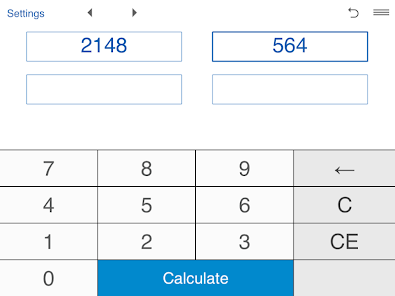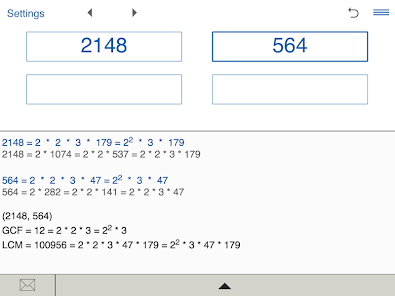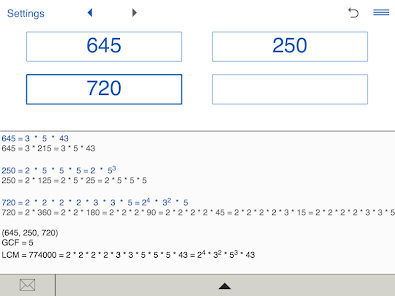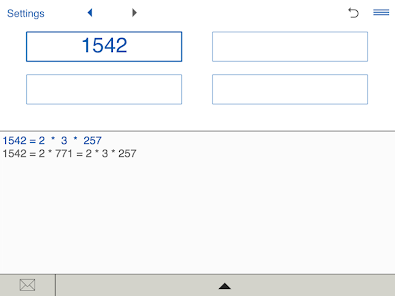A handy Prime Factor Calculator to find prime factors of any number.

Prime factorization of any number (up to 9 digits). With the Prime Factor Calculator you can factor up to four numbers at once. For prime numbers the calculator returns the number itself.

Prime factor calculator displays the prime factorization in standard and exponential forms.

In addition, the calculator calculates the GCF (Greatest Common Factor) and LCM (Least Common Multiple) of given numbers.

Prime Factor Calculator stores History list. So you can easily view and edit your recent calculations.

You can send results via email.

History list.
Updated on
Jul 30, 2020

## Data safety

Developers can show information here about how their app collects and uses your data. Learn more about data safetyNo information available